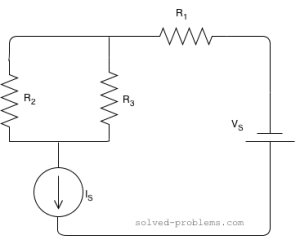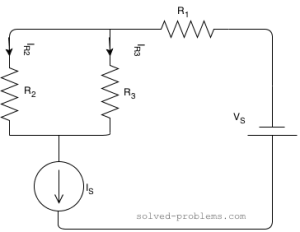Find current of resistors, use the current division rule.Suppose that $R_1=2 \Omega$, $R_2=4 \Omega$, $R_3=1 \Omega$, $I_S=5 A$ and $V_S=4 V$

Solution:
$R_2$ and $R_3$ are parallel. The current of $I_S$ is passing through them and it is actually divided between them. The branch with lower resistance has higher current because electrons can pass through that easier than the other branch. Using the current division rule, we get

$I_{R_2}=\frac{R_3}{R_2+R_3} \times I_S= \frac{1}{1+4} \times 5= 1 A$
$I_{R_3}=\frac{R_2}{R_2+R_3} \times I_S= \frac{4}{1+4} \times 5= 4 A$.
Note that $I_{R_2} because $R_2>R_3$.

What is the direction for $I_{R_2}$ and $I_{R_3}$? It is with the same direction as $I_S$:We have found $I_{R_2}$ and $I_{R_3}$, now we need to find $I_{R_1}$.

If you look at the circuit carefully, $R_1$, $V_S$ and $I_S$ are all in series. The bottom node of $I_S$ is connected to the bottom node of $V_S$ and there is no other component connected there. And the other node of $V_S$ is connected to $R_1$, again no other component here. This means that all current of $I_S$ should pass through $V_S$ and the same current must go through $R_1$. So, the current of $R_1$ can be easily found:
$I_{R_1}=I_S= 5 A$.

The direction is the same current as the current source direction:Now, tell me what is the voltage drop across the current source?

Hi! Yaz is here. I am passionate about learning and teaching. I try to explain every detail simultaneously with examples to ensure that students will remember them later too.

## Join the Conversation

1.2.3.4.5.6.1.Padu says:

Voltage drop is 14 V

1. KCV around the loop assuming the positive terminal of the current source to be the one at bottom:
$+V_{I_S}-R_3 \times I_{R_3}-R_1 \times I_{R_1}-V_S=0$

$V_{I_S}=R_3 \times I_{R_3}+R_1 \times I_{R_1}+V_S$
$V_{I_S}=1 \times 4+2 \times 5 +4=18 V$

If you label the voltage terminals of the current source the other way, you would get $-18 V$.
I think you forgot the voltage source.

2. VOLTEGE DROP IS 11V

3.abc says:

Sir please help me .I can't identify nodes in circuit tell me a wise definition that cover all aspects of node . If you illustrated this with a figure I'll be thankful.

4.avinesh says:

Good define thanku

5.nidhi says:

Sir,why are you not consider the mesh having the parallel combination of(4II1) for the calculation of voltage drop across current Is?

-Vs-R1*5-(4II1)*5-Vis=0
-4-2*5-(4\5)*5=Vis
-4-10-4=Vis
-18=Vis

7.lucas says:
8.Nazneen says: